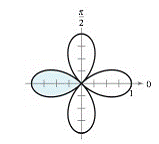Chapter 10.5, Problem 2E

Chapter
Section
Textbook Problem

# Area of a Polar Region In Exercises 3-6, write an integral that represents the area of the shaded region of the figure. Do not evaluate the integral. r = cos 2 θTo determine

To calculate: An integral that represents area of shaded region provided in the graph:Explanation

Given:

The polar equation is r=cos2θ and graph of area of shaded region as shown below:

Calculation:

Consider the function r=cos2θ

From the given graph petal of rose curve that lies between the radial lines.

The angle θ=3π4 and θ=5π4 as shown in below graph:

Area of shaded region bounded by graph of r=f(θ) between the radial lines θ=α and

θ=β is

A=12αβ[f(θ)]2.dθ=123π45π4[cos2θ]2

### Still sussing out bartleby?

Check out a sample textbook solution.

See a sample solution

#### The Solution to Your Study Problems

Bartleby provides explanations to thousands of textbook problems written by our experts, many with advanced degrees!

Get Started

#### In Exercises 7-10, find the slope of the line shown in each figure. 8.

Applied Calculus for the Managerial, Life, and Social Sciences: A Brief Approach

#### In problems 1-16, solve each equation. 7. Solve

Mathematical Applications for the Management, Life, and Social Sciences

#### Evaluate the integral. 0(5ex+3sinx)dx

Single Variable Calculus: Early Transcendentals

#### True or False: If and , then .

Study Guide for Stewart's Multivariable Calculus, 8th